8060

Mathematics Functions Level: Misc Level

sketch the graph of f (x)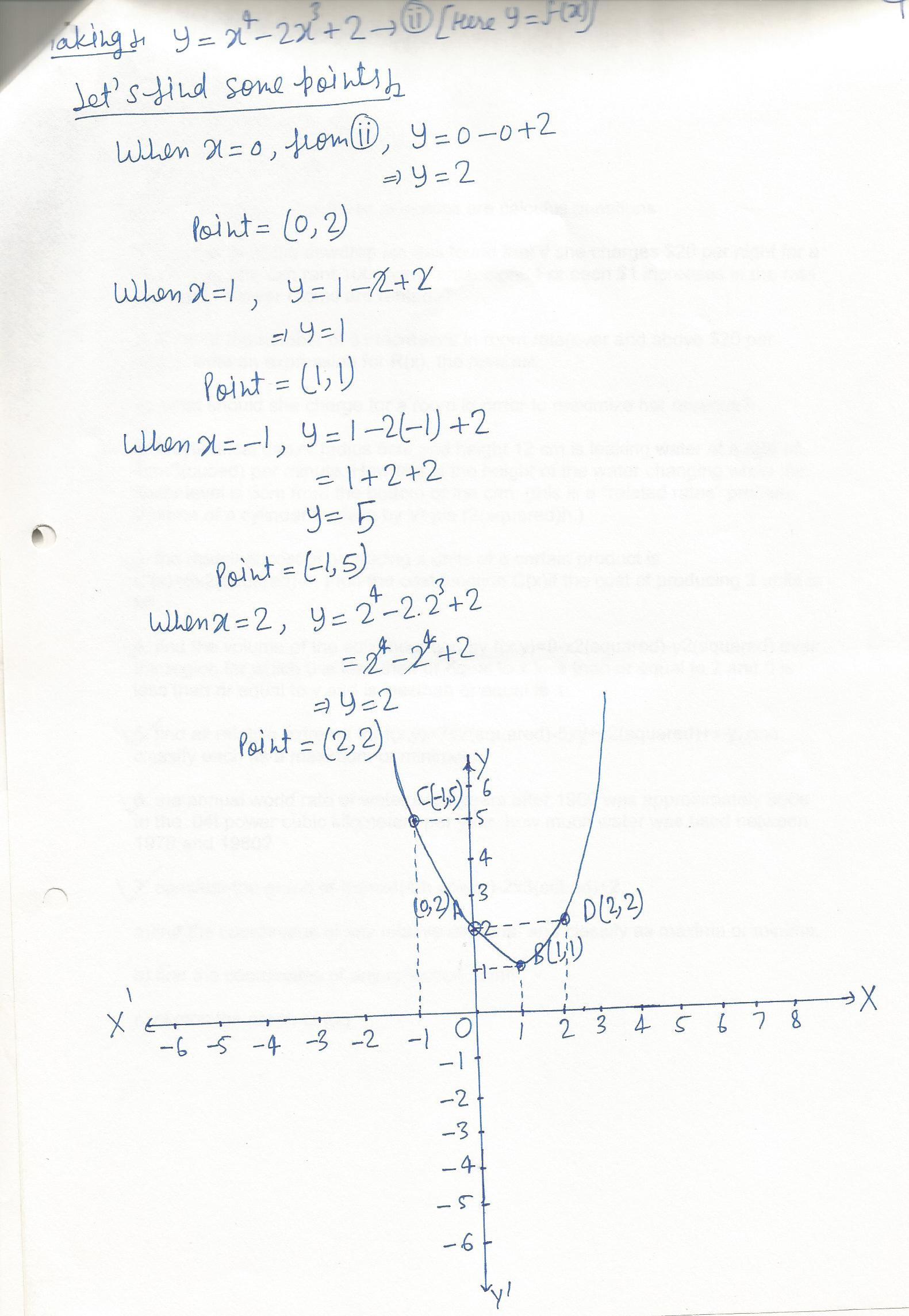8059

Mathematics Functions Level: Misc Level

find the coordinates of any reflection points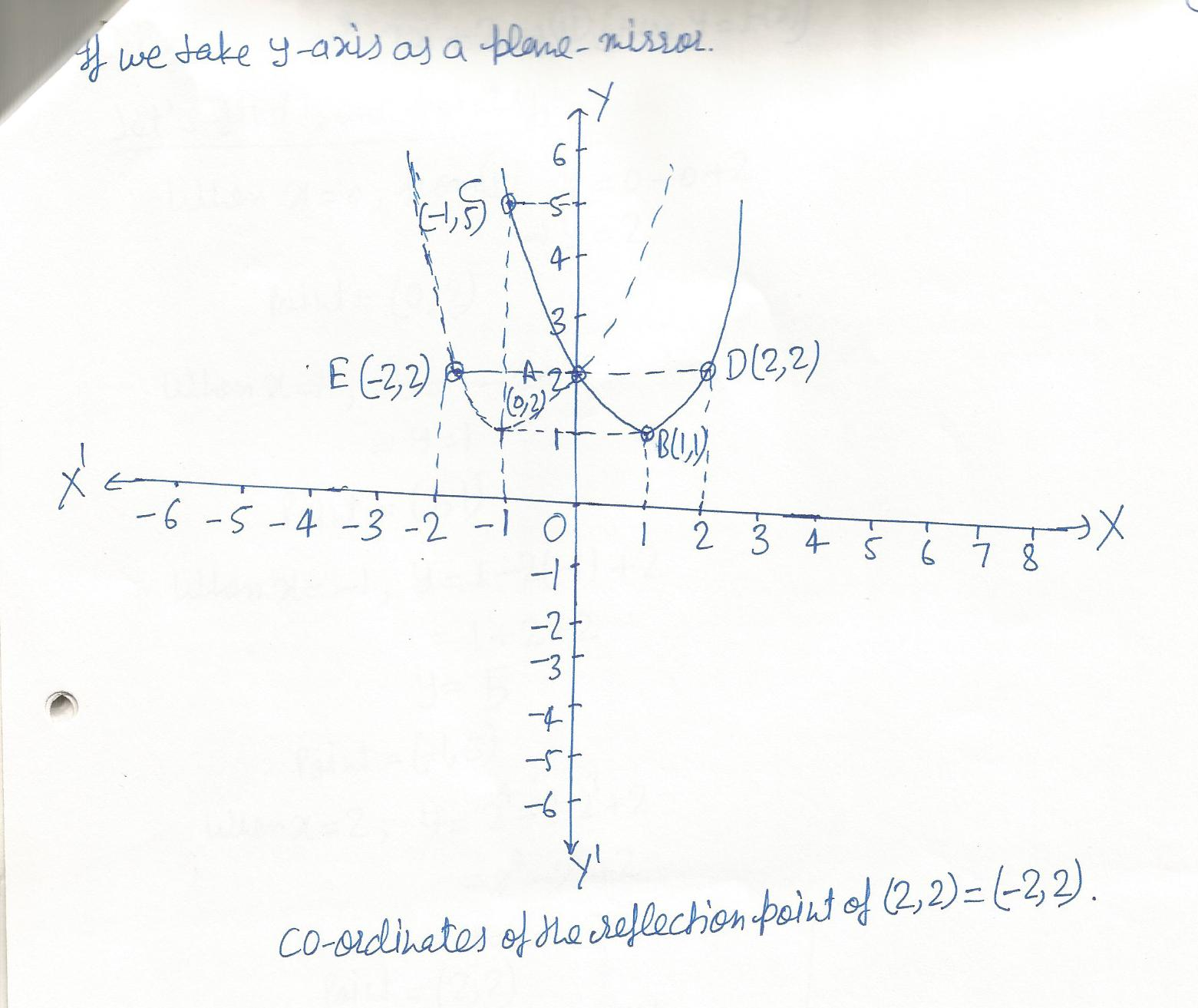8019

Mathematics Functions Level: Misc Level

Given f(x)=x^2-1 and g(x)=x^0.5, find the following;

a) f(g(x))

b) f (g(2))

c) g (f(x))

d) g(f(2))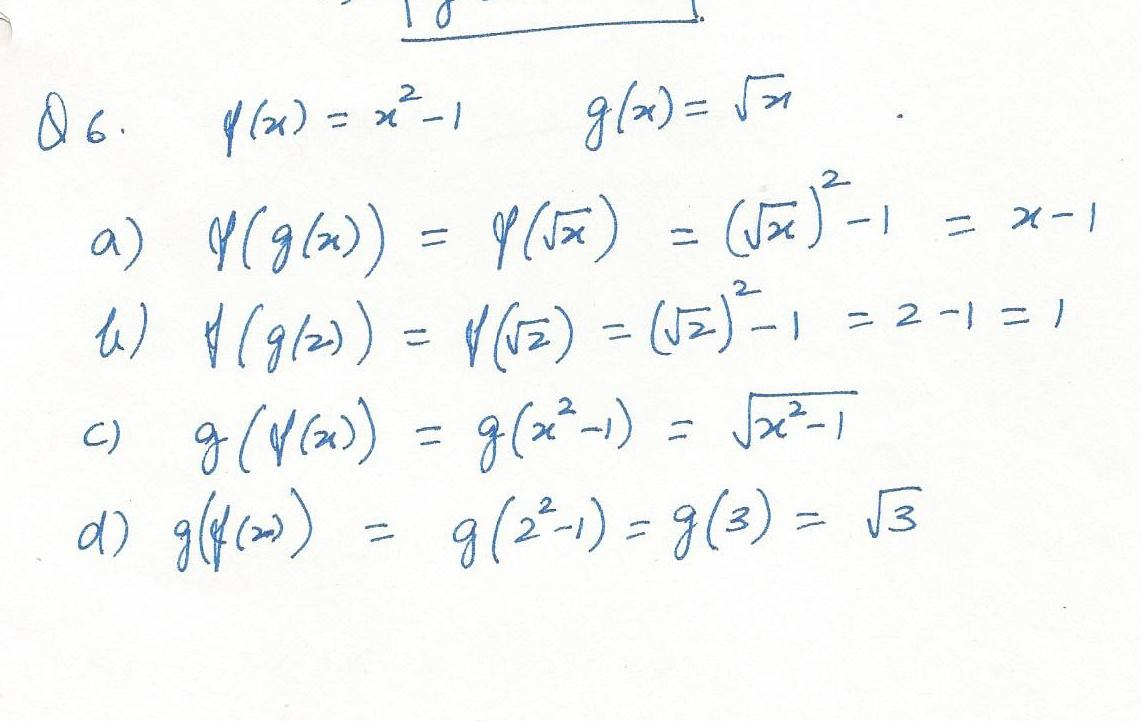8017

Mathematics Functions Level: Misc Level

Graph the function f(x)=x^2-3x-10 on the given grid. Find the x-intercepts and y-intercept.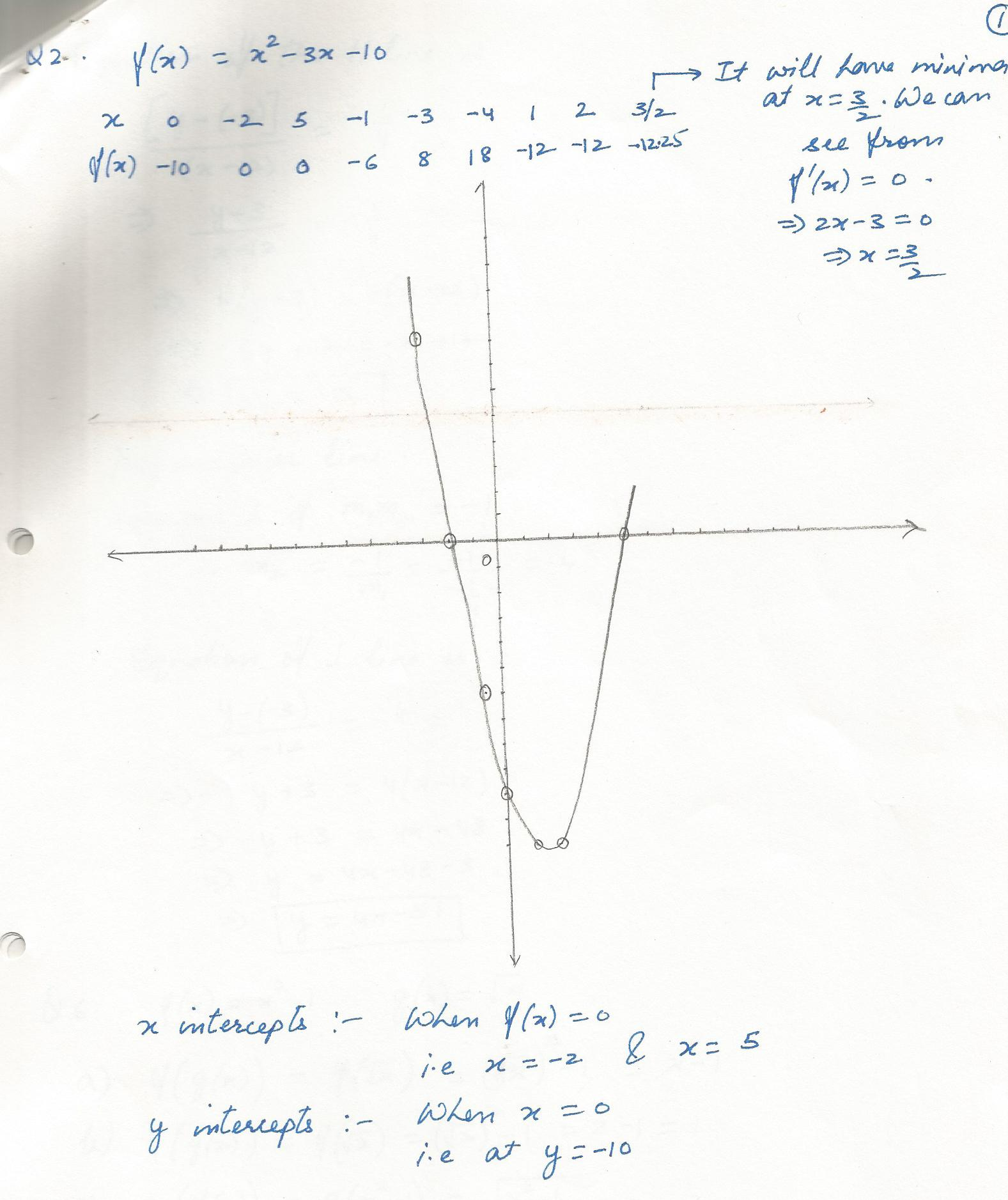7986

Mathematics Functions Level: Misc Level

Determine a linear function f(x), given f(2)=0.5;f(1)=-1.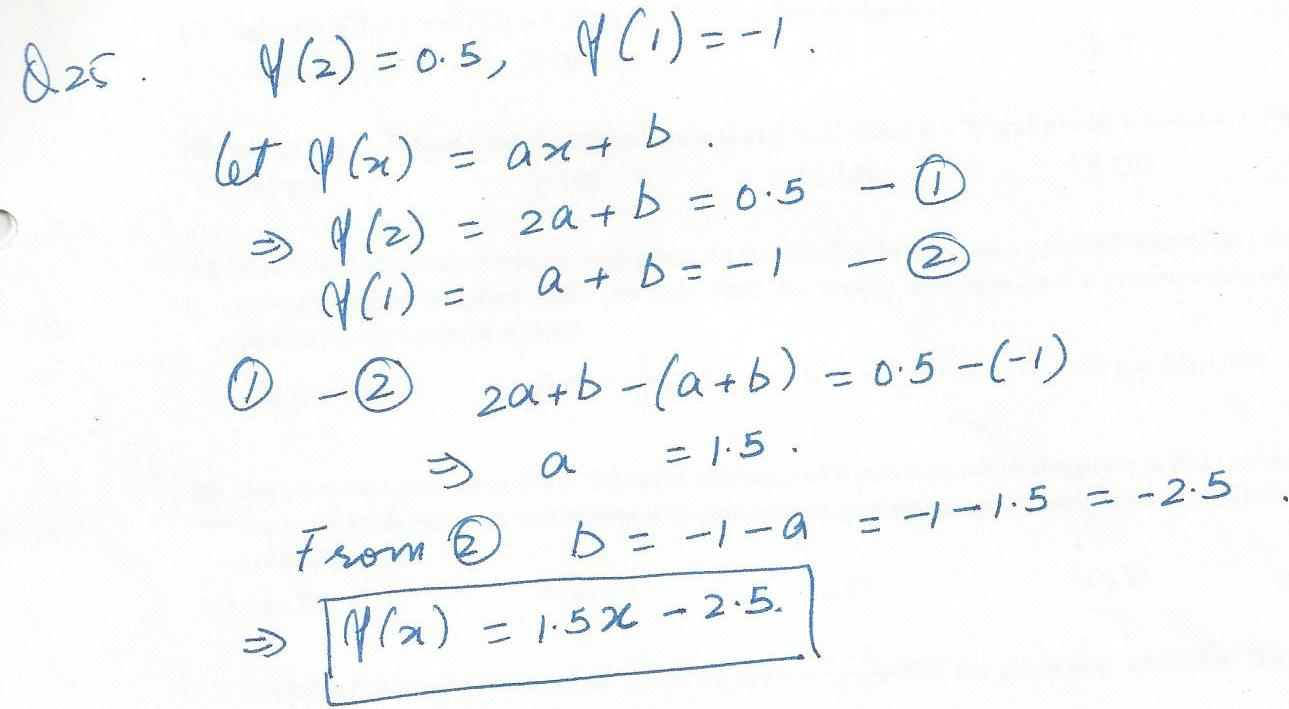7981

Mathematics Functions Level: Misc Level

Suppose f (0)=4 and f (3)=8. Find f(12) if f is a linear function.

A)6 B)20 C)24 D)-3 E)13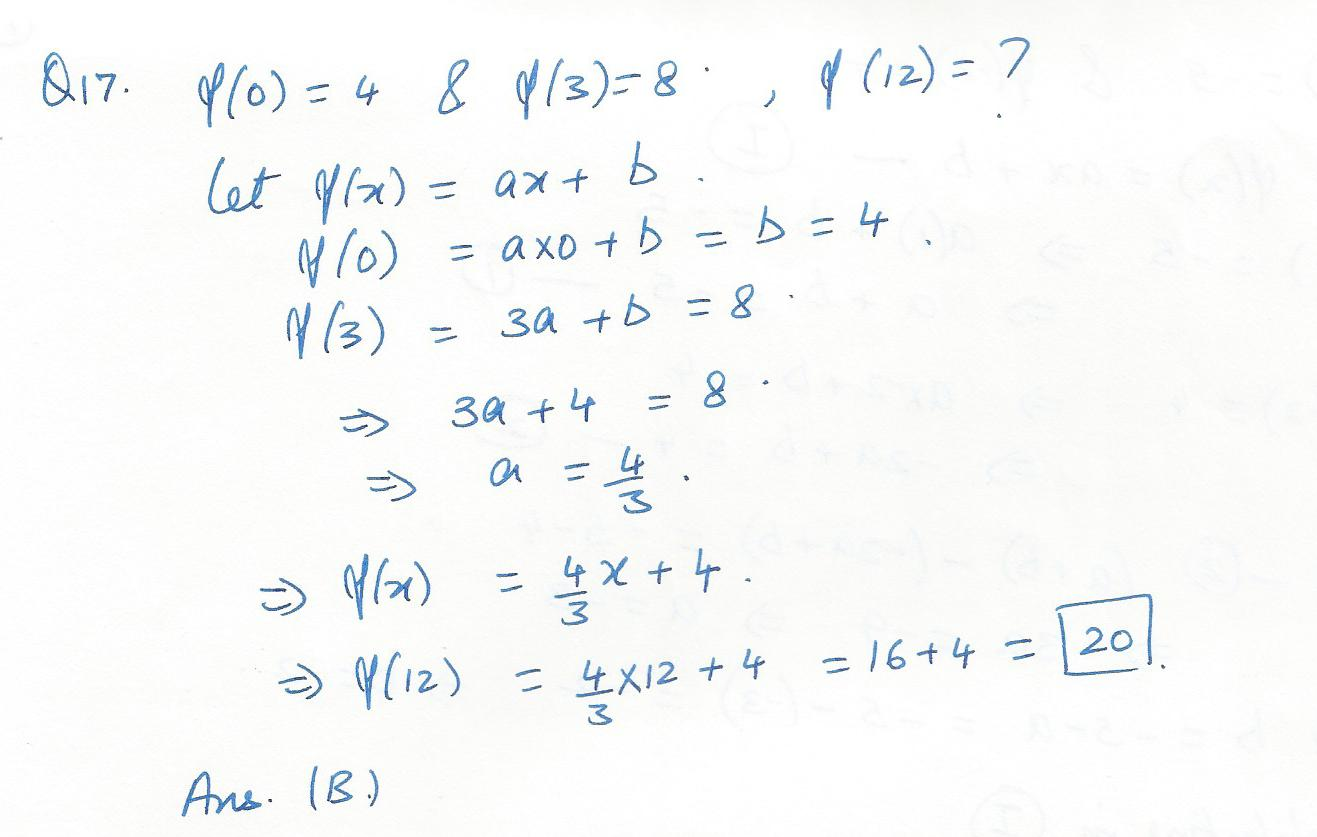7980

Mathematics Functions Level: Misc Level

Suppose f is a linear function with slope 2 and f (1)=3. Find f(2).

A)1 B)2 C)3 D)4 E)5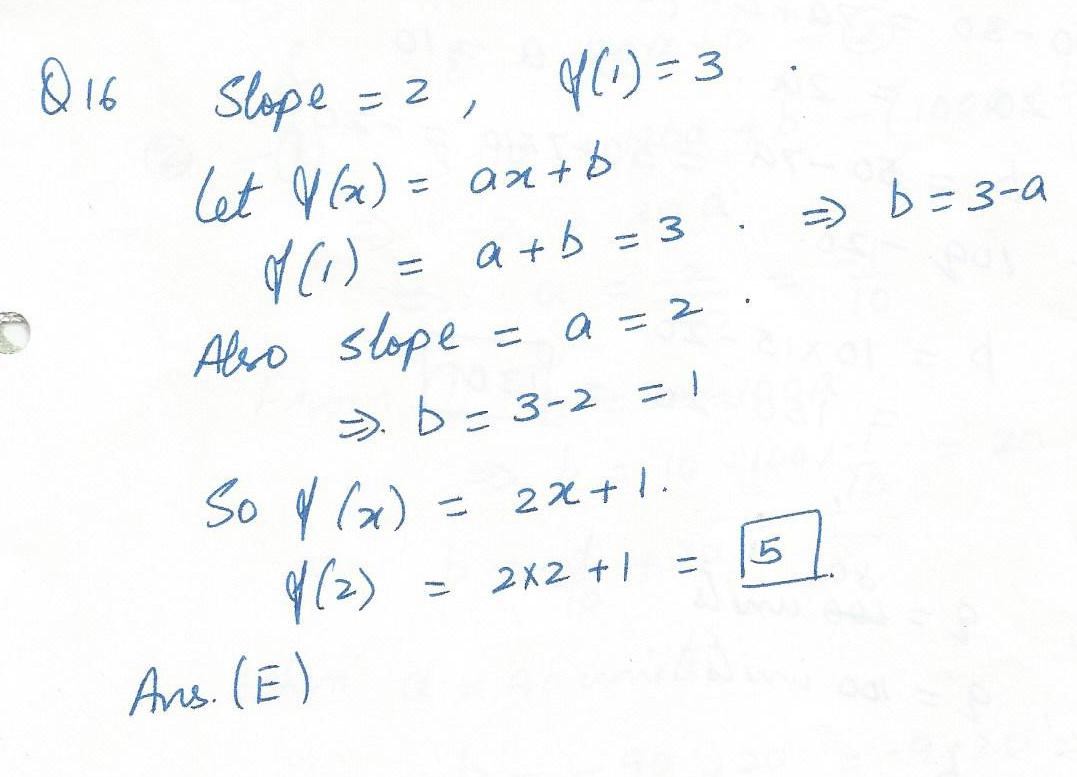7949

Mathematics Functions Level: Misc Level

Sketch the graph of y=log4x.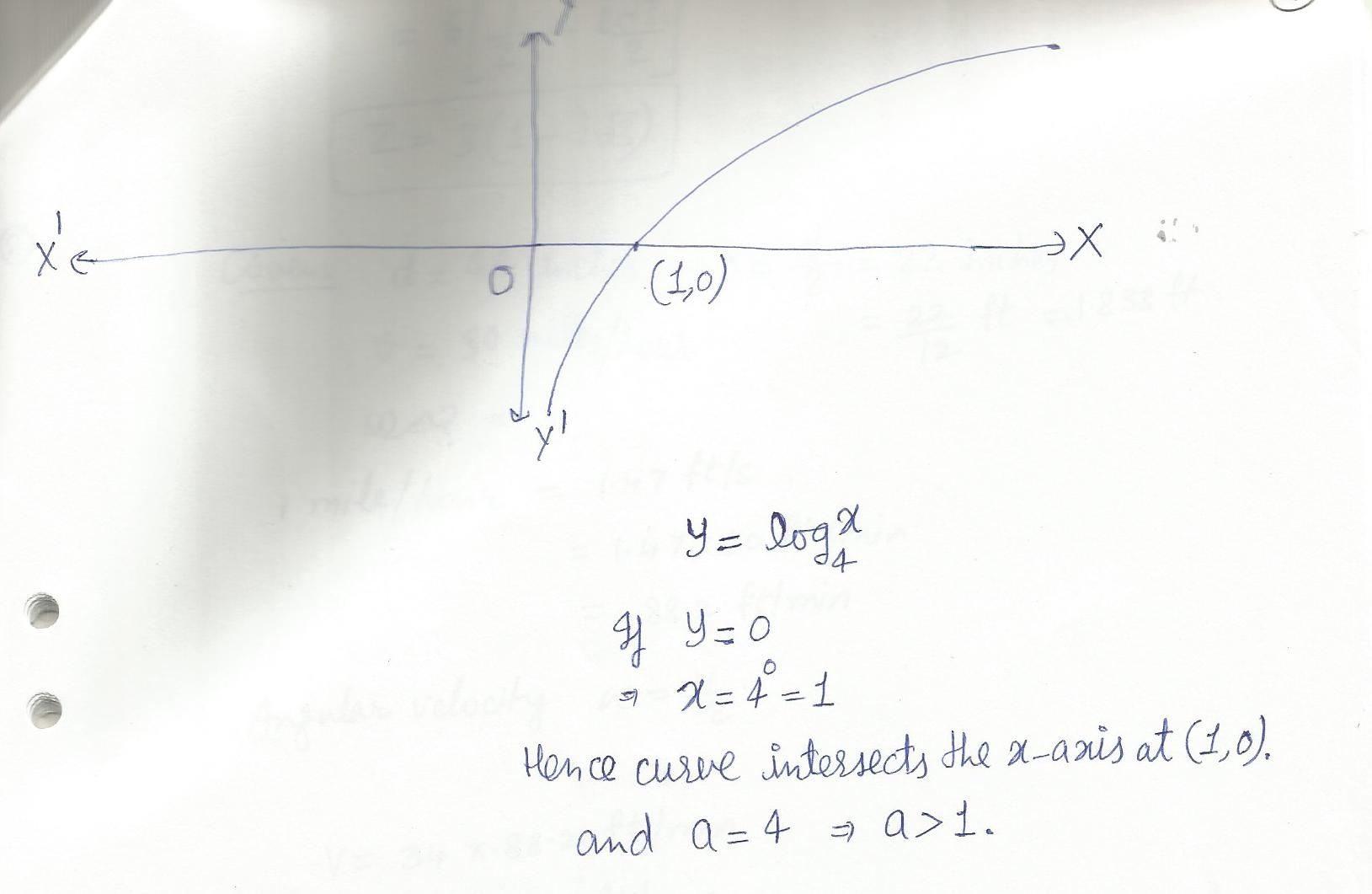Displaying 1-8 of 8 results.